# The Pentagonal Orthobirotunda

The pentagonal orthobirotunda is the 34th Johnson solid (J34). It has 30 vertices, 60 edges, and 32 faces (20 equilateral triangles and 12 pentagons).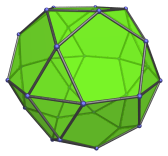The pentagonal orthobirotunda can be constructed by attaching two pentagonal rotundae to each other at their decagonal faces, such that their respective pentagons share edges with each other, and their triangles share edges with each other. The ortho- in the name refers to how the orientation of the top and bottom pentagons are aligned with each other. Joining the rotundae in gyro orientation produces the uniform icosidodecahedron instead. Thus, the icosidodecahedron may be thought of as a pentagonal gyrobirotunda.

The pentagonal orthobirotunda can be elongated by inserting a decagonal prism between its two halves, producing an elongated pentagonal orthobirotunda (J42).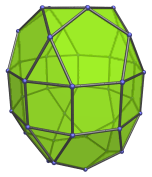## Projections

Here are some views of the pentagonal orthobirotunda from various angles:

Projection Description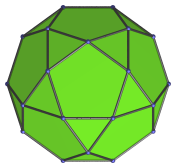Top view.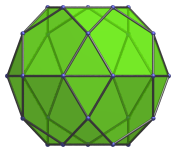Front view.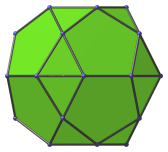Side view.

## Coordinates

The Cartesian coordinates of the pentagonal orthobirotunda with edge length 2 are:

• (−√((10+2√5)/5), 0, ±√((20+8√5)/5))
• (−√((5−√5)/10), ±φ, ±√((20+8√5)/5))
• ( √((5+2√5)/5), ±1, ±√((20+8√5)/5))
• ( √((20+8√5)/5), 0, ±√((10+2√5)/5))
• (−√((25+11√5)/10), ±φ, ±√((10+2√5)/5))
• ( √((5+√5)/10), ±φ2, ±√((10+2√5)/5))
• (±√(3+4φ), ±1, 0)
• (±√(2+φ), ±φ2, 0)
• (0, ±2φ, 0)

where φ = (1+√5)/2 is the Golden Ratio, approximately 1.61803.

Last updated 14 Sep 2020.